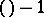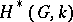# User:Rafael.greenblatt/sandbox

Jump to: navigation, search

(starting to modify "Exterior algebra")

Grassmann algebra, of a vector space $V$ over a field $k$

An associative algebra over $k$, the operation in which is denoted by the symbol $\wedge$, with generating elements $1,e_1,\ldots,e_n$ where $e_1,\ldots,e_n$ is a basis of $V$, and with defining relations

$$e_i \wedge e_j = - e_j \wedge e_i \qquad (i,j=1,\ldots,n), \qquad e_i \wedge e_i = 0,$$

$$1 \wedge e_i = e_i \wedge 1 = e_i \qquad (i=1,\ldots,n), \qquad \ \wedge 1 = 1.$$

The exterior algebra does not depend on the choice of the basis and is denoted by $\wedge V$. The subspace $\wedge^r V$ ($r=0,1,\ldots$) in $\wedge V$ generated by the elements of the form $e_{i_1} \wedge \ldots \wedge e_{i_r}$ is said to be the $r$-th exterior power of the space $V$. The following equalities are valid: $\dim \wedge^r V = () = C_n^r$, $r=0,\ldots,n$, $\wedge^r V =0$, $r>n$. In addition, $v \wedge u = (-1)^{rs}u \wedge v$ if $u \in \wedge^r V$, $v \in \wedge^s V$. The elements of the space $\wedge^r V$ are said to be $r$-vectors; they may also be regarded as skew-symmetric $r$-times contravariant tensors in $V$ (cf. Exterior product).

$r$-vectors are closely connected with $r$-dimensional subspaces in $V$: Linearly independent systems of vectors $x_1,\ldots,x_r$ and $y_1,\ldots,y_r$ of $V$ generate the same subspace if and only if the $r$-vectors $x_1\wedge \ldots \wedge x_r$ and $y_1\wedge \ldots \wedge y_r$ are proportional. This fact served as one of the starting points in the studies of H. Grassmann , who introduced exterior algebras as the algebraic apparatus to describe the generation of multi-dimensional subspaces by one-dimensional subspaces. The theory of determinants is readily constructed with the aid of exterior algebras. An exterior algebra may also be defined for more general objects, viz. for unitary modules $M$ over a commutative ring $A$ with identity . The $r$-th exterior power $\wedge^r M$, $r>0$, of a module $M$ is defined as the quotient module of the $r$-th tensor power of this module by the submodule generated by the elements of the form $x1 \otimes \ldots \otimes x_r$, where $x_i \in M$ and $x_j=x_k$ for certain $j \ne k$. The exterior algebra for $M$ is defined as the direct sum $\wedge M = \bigoplus_{r \ge 0}\wedge^r M$, where $\wedge^0 M = A$, with the naturally introduced multiplication. In the case of a finite-dimensional vector space this definition and the original definition are identical. The exterior algebra of a module is employed in the theory of modules over a principal ideal ring .

The Grassmann (or Plücker) coordinates of an $r$-dimensional subspace $L$ in an $n$-dimensional space $V$ over $k$ are defined as the coordinates of the $r$-vector in $V$ corresponding to $L$, which is defined up to proportionality. Grassmann coordinates may be used to naturally imbed the set of all-dimensional subspaces ininto the projective space of dimension, where it forms an algebraic variety (called the Grassmann manifold). Thus one gets several important examples of projective algebraic varieties .

Exterior algebras are employed in the calculus of exterior differential forms (cf. Differential form) as one of the basic formalisms in differential geometry , . Many important results in algebraic topology are formulated in terms of exterior algebras.

E.g., ifis a finite-dimensional-space (e.g. a Lie group), the cohomology algebraofwith coefficients in a fieldof characteristic zero is an exterior algebra with odd-degree generators. Ifis a simply-connected compact Lie group, then the ring, studied in-theory, is also an exterior algebra (over the ring of integers).

How to Cite This Entry:
Rafael.greenblatt/sandbox. Encyclopedia of Mathematics. URL: http://encyclopediaofmath.org/index.php?title=Rafael.greenblatt/sandbox&oldid=20503